Welcome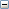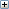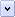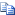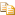IGetControlPoints Method (ISplineParamData)

Gets the coordinates of all of the control points of the spline.

# .NET Syntax

Visual Basic (Declaration)
```Function IGetControlPoints( _
ByVal Count As System.Integer, _
ByRef ControlPoints As System.Double _
) As System.Boolean```
Visual Basic (Usage)
```Dim instance As ISplineParamData
Dim Count As System.Integer
Dim ControlPoints As System.Double
Dim value As System.Boolean

value = instance.IGetControlPoints(Count, ControlPoints)```
C#
```System.bool IGetControlPoints(
System.int Count,
out System.double ControlPoints
)```
C++/CLI
```System.bool IGetControlPoints(
&   System.int Count,
&   [Out] System.double ControlPoints
) ```

#### Parameters

Count
Size of ControlPoints array
ControlPoints
• in-process, unmanaged C++: Pointer to an array of doubles (see Remarks)
• VBA, VB.NET, C#, and C++/CLI: Not supported

• #### Return Value

True if successful, false if not

# Remarks

Before calling this method, call ISplineParamData::ControlPointsCount to populate the Count parameter.

Control points determine the shape of a spline or curve. Starting with a set of control points, all other points of a spline can be calculated. A given point on the spline is calculated using the polynomial basis functions of its nearest control points. The knot vector determines which control point basis functions are in force for calculating each point along a curve.

The size of the ControlPoints array is based on the curve dimension:

• if dimension = 2 then each control point has 2 doubles ( x, y )

• if dimension = 3 then each control point has 3 doubles ( x, y, z )

• if dimension = 4, and the curve is closed or periodic, then each control point has 4 doubles ( x, y, z, w ), where w = weight

• if dimension = 4, and the curve is open or non-periodic, then each control point has 4 doubles ( w*x, w*y, w*z, w ), where w = weight

• Size of ControlPoints array = control points count * dimension

# Availability

SOLIDWORKS 2011 FCS, Revision Number 19.0

Provide feedback on this topic

* Required

 *Email: Subject: Feedback on Help Topics Page: IGetControlPoints Method (ISplineParamData) *Comment: * I acknowledge I have read and I hereby accept the privacy policy under which my Personal Data will be used by Dassault Systèmes

Print Topic

Select the scope of content to print:

x

We have detected you are using a browser version older than Internet Explorer 7. For optimized display, we suggest upgrading your browser to Internet Explorer 7 or newer.

Never show this message again
x

Web Help Content Version: API Help (English only) 2016 SP05

To disable Web help from within SOLIDWORKS and use local help instead, click Help > Use SOLIDWORKS Web Help.

To report problems encountered with the Web help interface and search, contact your local support representative. To provide feedback on individual help topics, use the “Feedback on this topic” link on the individual topic page.